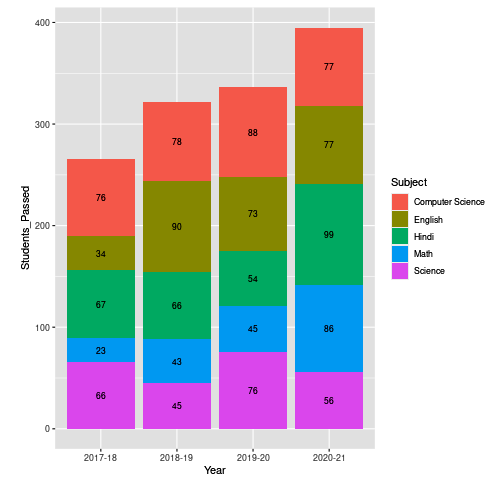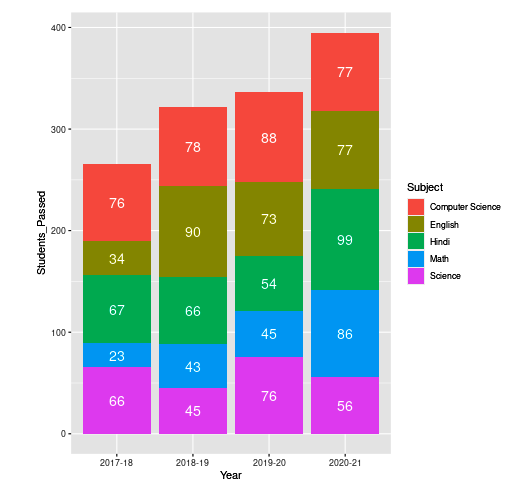Related Articles

# Showing data values on stacked bar chart in ggplot2 in R

• Last Updated : 29 Jun, 2021

In this article, you’ll learn how to show data values on a stacked bar chart in ggplot2 in R Programming Language.

To show the data into the Stacked bar chart you have to use another parameter called geom_text().

Syntax:

geom_text(size, position = position_stack(vjust = value), colour)

Here the size represents the size of the font that will appear on the plot and position_stack() will automatically add values to the plot at their respective positions.

Example 1:

## R

 `# Creating the Data``Subject = ``c``(``rep``(``c``(``"Hindi"``, ``"English"``, ``"Math"``, ``"Science"``,``                  ``"Computer Science"``), times = 4))`` ` `Year = ``c``(``rep``(``c``(``"2017-18"``, ``"2018-19"``, ``"2019-20"``,``               ``"2020-21"``), each = 5))`` ` `Students_Passed = ``c``(67,34,23,66,76,66,90,43,45,78,54,73,``                    ``45,76,88,99,77,86,56,77)`` ` `# Passing the Data to DataFrame``Students_Data = ``data.frame``(Subject,Year,Students_Passed)`` ` `# loading the Library``library``(ggplot2)`` ` `# Plotting the Data in ggplot2``ggplot``(Students_Data, ``aes``(x = Year, y = Students_Passed, ``                          ``fill = Subject, label = Students_Passed)) +``geom_bar``(stat = ``"identity"``) + ``geom_text``(``  ``size = 3, position = ``position_stack``(vjust = 0.5))   `

Output:It is also possible to change the color of data values using geom_text() itself. For this just pass the font color to the color attribute.

Example 2:

## R

 `# Creating the Data``Subject = ``c``(``rep``(``c``(``"Hindi"``, ``"English"``, ``"Math"``, ``"Science"``,``                  ``"Computer Science"``), times = 4))``Year = ``c``(``rep``(``c``(``"2017-18"``, ``"2018-19"``, ``"2019-20"``,``               ``"2020-21"``), each = 5))``Students_Passed = ``c``(67,34,23,66,76,66,90,43,45,78,54,``                    ``73,45,76,88,99,77,86,56,77)`` ` `# Passing the Data to DataFrame``Students_Data = ``data.frame``(Subject,Year,Students_Passed)`` ` `# loading the Library``library``(ggplot2)`` ` `# Plotting the Data in ggplot2``ggplot``(Students_Data, ``aes``(x = Year, y = Students_Passed, ``                          ``fill = Subject, label = Students_Passed)) +``geom_bar``(stat = ``"identity"``) + ``geom_text``(``  ``size = 5, position = ``position_stack``(vjust = 0.5),colour = ``"white"``)   `

OutputMy Personal Notes arrow_drop_up